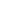| lesson material | material summary | questions and answers | definitions | types and examples | other information | materi pelajaran | ringkasan materi | pertanyaan dan jawaban | definisi | jenis-jenis dan contoh-contoh | informasi lainnya |

# Get to know HTML5 Canvas for Graphic Programming and Games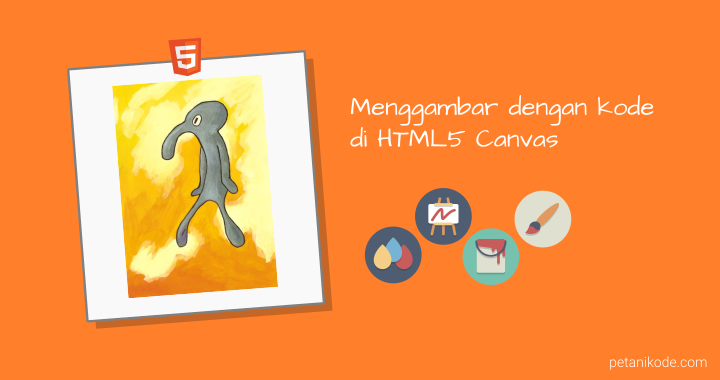Before the arrival of HTML5, games and other multimedia elements were presented in the browser using Flash.
Many Flash-based games can be played through a browser. Even Flash is also used to play videos.
As far as I remember ... without Flash Player, we can't play videos on Youtube.
But everything changed after the arrival of HTML5 ... 1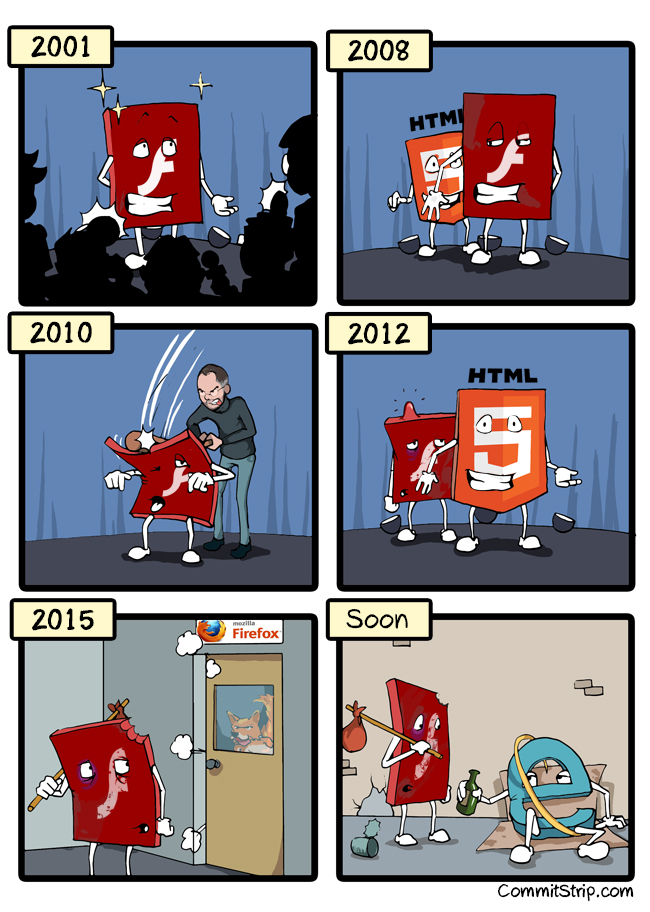HTML5 brings many new elements that replace Flash tasks in the browser. One of them is canvas .

## What is Canvas?

In the real world, canvas is often used for drawing and painting. Usually using pencils and paint.
In programming, canvas is an element that can be drawn with code. 2
Canvas is a new element in HTML5 for drawing (rendering) graphics, images, and text.

## How to Make Canvas in HTML

Canvas can be made with tags `<canvas>`, don't forget to give the size of the height and width.
``````<canvas id="myCanvas" width="640" height="480" style="border:1px solid #000000;">
</canvas>
``````
We give a little style `style="border:1px solid #000000;"`to make a border.
Next we have to use the DOM API to manipulate the canvas , usually done like this:
``````var canvas = document.getElementById("myCanvas");
var ctx = canvas.getContext("2d");
``````
Function `document.getElementById("myCanvas")`to select HTML elements with id `myCanvas`.
Next the variable `ctx`(context) is an object from canvas that we can use to draw.
Sample complete code:
``````<!DOCTYPE html>
<html>
<meta charsetgoyang dombret="utf-8">
<title>Tutorial HTML 5 Canvas</title>
<body>

<canvas id="myCanvas" width="640" height="480" style="border:1px solid #000000;">
</canvas>

<script type="text/javascript">
var canvas = document.getElementById("myCanvas");
var ctx = canvas.getContext("2d");
</script>

</body>
</html>
``````
Now we have an empty canvas ready to be drawn ...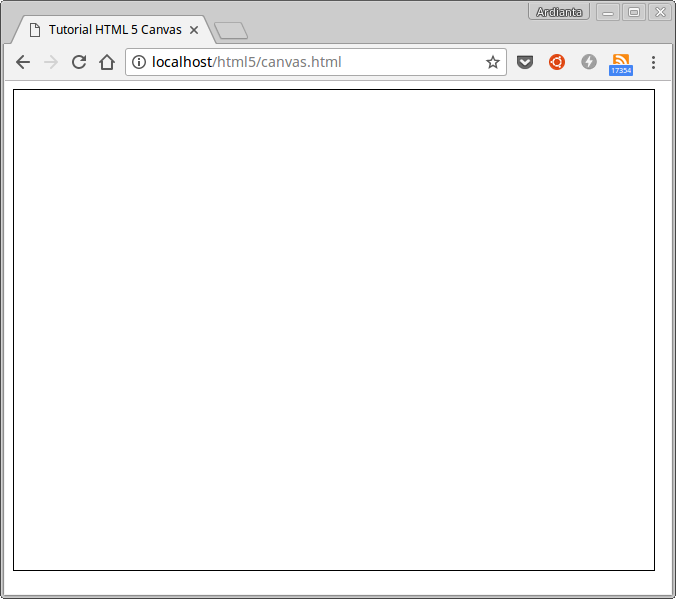Prepare your imagination and start using the code.

## Draw on Canvas

If in the real world we use pencils to draw, then on HTML5 canvas uses objects `ctx`.
`ctx`We can think of objects as pencils. This object has several methods for drawing on canvas such as `fillRect()``arc()``fillText()`, etc.
Now to draw with these methods, we must determine the coordinates of the point `x`and `y`its.
Because the canvas consists of a collection of pixels that form an inverted cartesius diagram.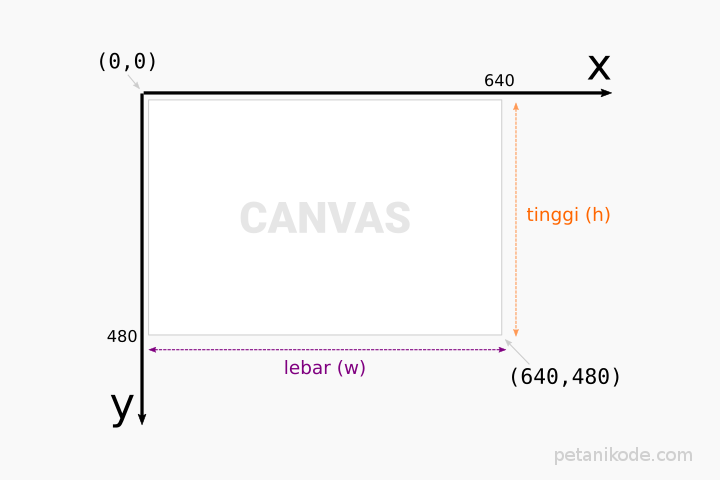### How to draw points on canvas

First we will try to draw a point.
In art, a point is the smallest part of an object that occupies a space. Whereas in geometric mathematics, dots are objects `0`that do not have length, width, and height. 3
But in a computer the point is the size of 1 pixel from the screen.
To draw a point on the canvas, we can use a method `fillRect()` with a size of 1x1 pixels.
``````ctx.fillRect(x,y,1,1);
``````
Variables `x`and `y`we replace them with coordinate values, for example we want to draw a point on coordinates `(10,10)`.
``````ctx.fillRect(10,10,1,1);
``````
Let's try in the code:
``````<!DOCTYPE html>
<html>
<meta charset="utf-8">
<title>Tutorial HTML 5 Canvas</title>
<body>

<canvas id="myCanvas" width="640" height="480" style="border:1px solid #000000;">
</canvas>

<script type="text/javascript">
var canvas = document.getElementById("myCanvas");
var ctx = canvas.getContext("2d");

// gambar titik pada koordinat (10,10) dengan ukuran 1x1 px
ctx.fillRect(10,10,1,1);
</script>

</body>
</html>
``````
The result: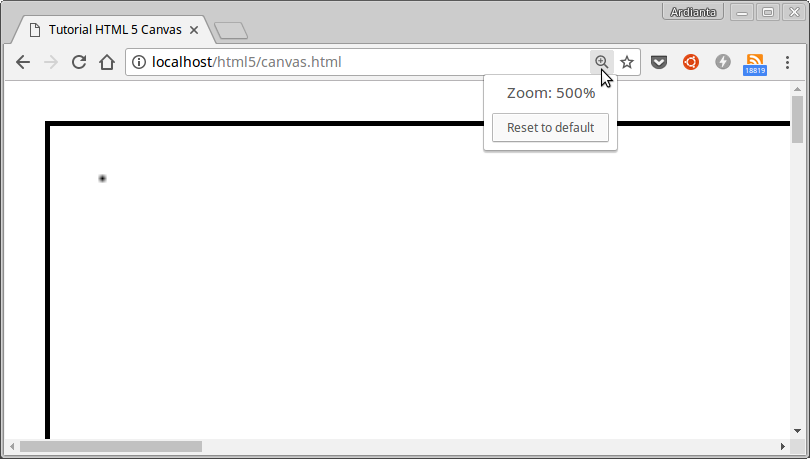(Make an increase of up to 500% to see it)

### How to Draw a Line on Canvas

A line is a collection of connected points. There are four functions that we need to make a line.
1. `ctx.beginPath()` to make a new line.
2. `ctx.moveTo(x,y)` to determine the starting point.
3. `ctx.lineTo(x,y)` to determine the end point.
4. `ctx.stroke()` to draw the line.
Let's try ...
Suppose we want to draw a line from point `(10,10)`to point `(50,50)`.
``````<!DOCTYPE html>
<html>
<meta charset="utf-8">
<title>Tutorial HTML 5 Canvas</title>
<body>

<canvas id="myCanvas" width="640" height="480" style="border:1px solid #000000;">
</canvas>

<script type="text/javascript">
var canvas = document.getElementById("myCanvas");
var ctx = canvas.getContext("2d");

ctx.beginPath(); // mulai menggmabar
ctx.moveTo(10,10);
ctx.lineTo(50,50);
ctx.stroke();
</script>

</body>
</html>
``````
The result: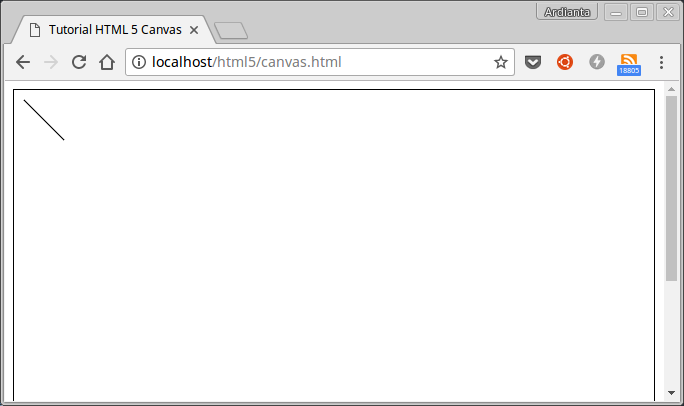### How to Draw Square on Canvas

There are three functions used to draw a square on a canvas :
1. `ctx.strokeRect(x,y,w,h)`square with an outline .
2. `ctx.fillRect(x,y,w,h)` square with color finish (default is black).
3. `ctx.clearRect(x,y,w,h)` transparent square to delete.
As usual, we must provide coordinates `x`and `y`While the value `w`and `h`for width and height.
Let's try:
``````<!DOCTYPE html>
<html>
<meta charset="utf-8">
<title>Tutorial HTML 5 Canvas</title>
<body>

<canvas id="myCanvas" width="640" height="480" style="border:1px solid #000000;">
</canvas>

<script type="text/javascript">
var canvas = document.getElementById("myCanvas");
var ctx = canvas.getContext("2d");

ctx.strokeRect(10,10,100,100);
ctx.fillRect(120,10,100,100);

</script>

</body>
</html>
``````
The result: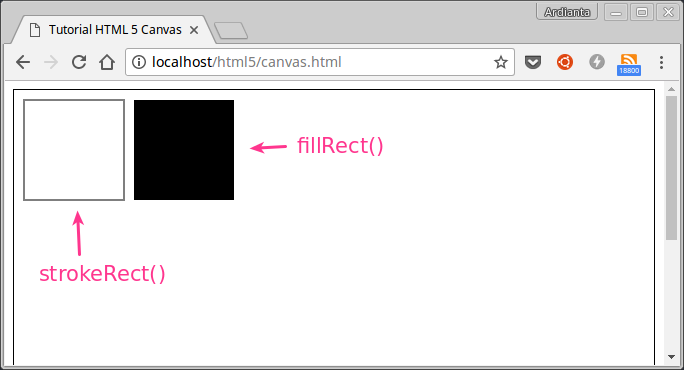Then what about the example `clearRect()`?
Method `clearRect()`used to delete images.
Example: We want to delete at the point of `(5,5)`arrival `(20,20)`.
``````var canvas = document.getElementById("myCanvas");
var ctx = canvas.getContext("2d");

ctx.strokeRect(10,10,100,100);
ctx.clearRect(5,5,20,20);
ctx.fillRect(120,10,100,100);
``````
Then the result: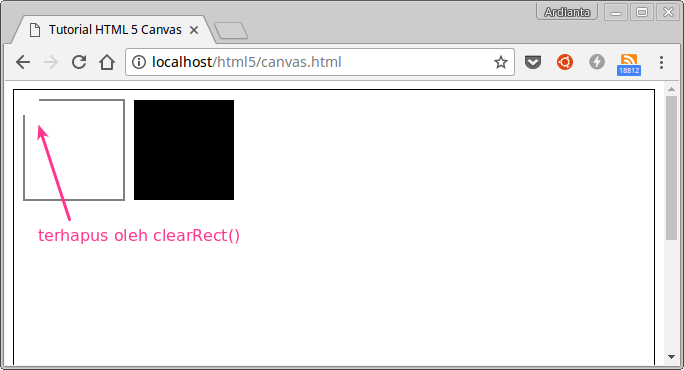### How to Draw Triangles on Canvas

Triangle does not have tesendiri method such as square (rectangle) . However, that does not mean we cannot draw it.
Triangles can be drawn using functions / methods from lines.
Example:
``````var canvas = document.getElementById("myCanvas");
var ctx = canvas.getContext("2d");

ctx.beginPath(); // mulai menggmabar
ctx.moveTo(100, 100); // titik awal
ctx.lineTo(100, 300); // titik ke-2
ctx.lineTo(300, 300); // titik ke-3
ctx.closePath(); // titik ke-3 ke titik awal

// buat garis
ctx.stroke();
``````
Then the result: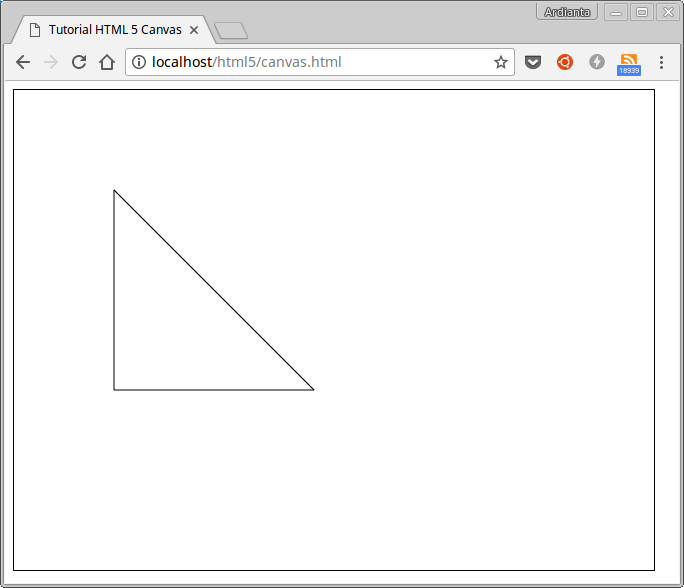Note : there is a function `ctx.closePath()`, the task is to draw a line from the last point to the starting point.
First line drawing from the starting point to the second point:
``````ctx.moveTo(100, 100);
ctx.lineTo(100, 300);
``````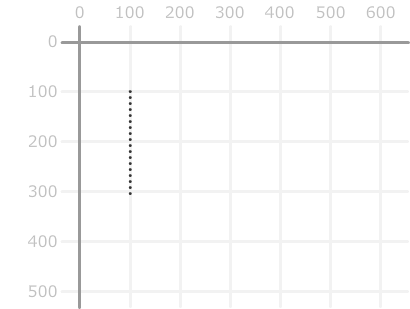Then draw a line from the 2nd point to point 3:
``````ctx.lineTo(300, 300);
``````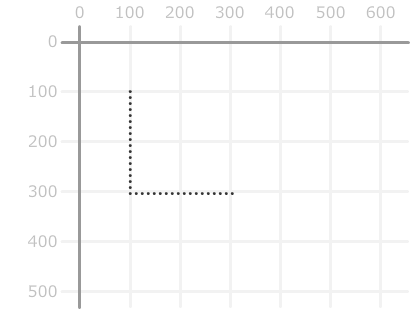Finally, draw a line from the 3rd point to the starting point:
``````ctx.closePath();
``````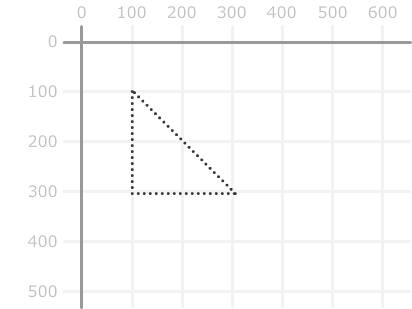Then be a triangle ...### How to Draw a Circle on Canvas

Circles are drawn by method `arc()`This method has 5 parameters that must be given.
``````ctx.arc(x, y, r, sudutAwal, sudutAkhir, arah);
``````
Paramter:
• `x`to determine coordinates `x`;
• `y`to determine coordinates `y`;
• `r` to determine the radius of the circle;
• `sudutAwal`initial angle with radians ( `0`radians starting at 3 o'clock);
• `sudutAkhir` final angle with radians;
• `arah` (optional) to determine the direction of drawing a circle:
• if given a value `true`it will draw counterclockwise.
• if `false`it will draw clockwise.
• The default value is `false`.
Understand…?
Let's try:
``````var canvas = document.getElementById("myCanvas");
var ctx = canvas.getContext("2d");

ctx.beginPath();
ctx.arc(95, 50, 40, 0, 2*Math.PI);
ctx.stroke();
``````
The result: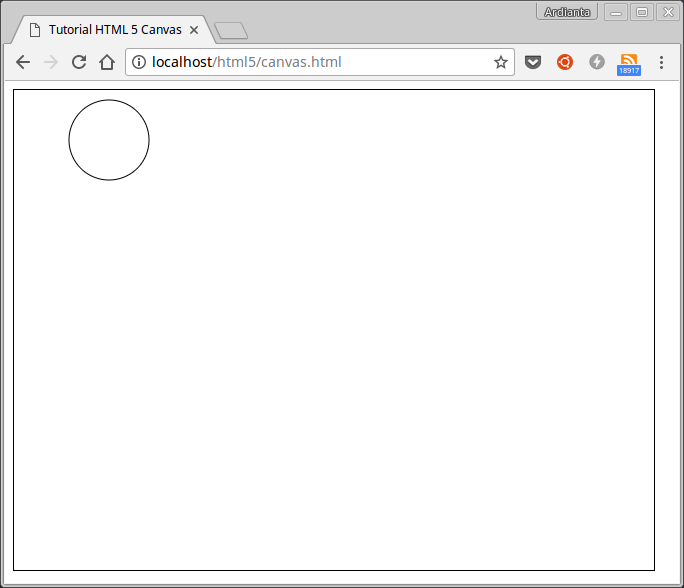Explanation :
First we start preaching with `beginPath()`, always use this function to start the news.
The second uses a circle with functions `arc()`with specified parameters. The circle is drawn at the point `(95, 50)`with the radius of the `40`pixel.
Start to be drawn from 3 o'clock ( `0`radians) to return to 3 o'clock ( `2*π`radians). 4It's easy?

### How to Insert Text on Canvas

There are two functions for creating text:
1. `strokeText()`for text with only outlines .
2. `fillText()` for text with color (default is black).
The function has three parameters that must be given:
``````ctx.fillText("Teks yang akan dibuat", x, y);
``````
Let's try:
``````var canvas = document.getElementById("myCanvas");
var ctx = canvas.getContext("2d");

ctx.font = "30px arial";
ctx.fillText("Terima kasih sudah berkunjung ke:",10,50);

ctx.font = "64px Arial";
ctx.strokeText("PETANIKODE.com",10,120);
``````
The result: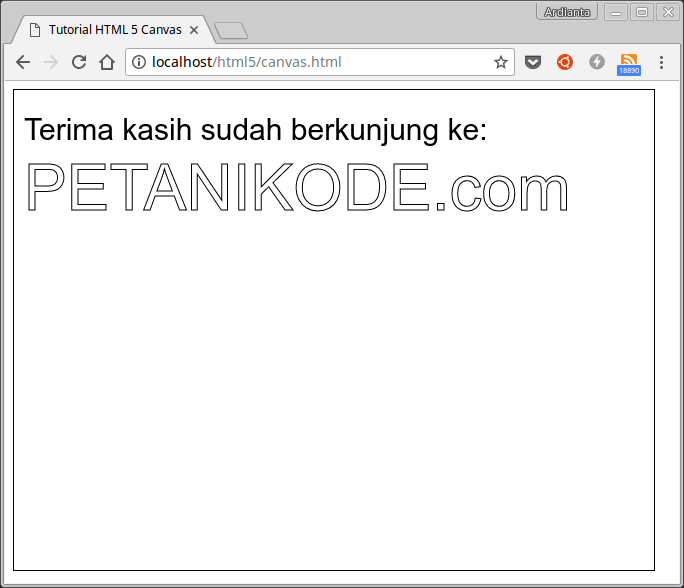There are filling attributes `font`in the code above.
``````ctx.font = "64px Arial";
``````
Its function is to determine the font type and size. The value is like the attribute value `font`in CSS.

### How to Render Images on Canvas

Finally, we will try to render the image to the canvas. To do this, we need an image and use the function `drawImage()`to render it to the canvas.
``````drawImage(img, x, y);
``````
We will use images from W3Schools: img_the_scream.jpg .
First, please create an element `<img>`:
``````<p>Gambar yang akan di-render:</p>
<img id="scream" src="https://www.w3schools.com/html/img_the_scream.jpg" alt="The Scream" width="220" height="277">
``````
Then make the rendering button:
``````<p><button onclick="renderImage()">Render Image</button></p>
``````
Finally make a function `renderImage()`:
``````function renderImage() {
var canvas = document.getElementById("myCanvas");
var ctx = canvas.getContext("2d");

// ambil gambar dari elemen <img>
var img = document.getElementById("scream");

// render ke Canvas
ctx.drawImage(img,10,10);
}
``````
The complete code:
``````<!DOCTYPE html>
<html>
<meta charset="utf-8">
<title>Tutorial HTML 5 Canvas</title>
<body>

<p>Gambar yang akan di-render:</p>
<img id="scream" src="https://www.w3schools.com/html/img_the_scream.jpg" alt="The Scream" width="220" height="277">

<p><button onclick="renderImage()">Render Image</button></p>

<canvas id="myCanvas" width="640" height="480" style="border:1px solid #000000;">
</canvas>

<script type="text/javascript">
function renderImage() {
var canvas = document.getElementById("myCanvas");
var ctx = canvas.getContext("2d");

// ambil gambar dari elemen <img>
var img = document.getElementById("scream");

// render ke Canvas
ctx.drawImage(img,10,10);
}
</script>

</body>
</html>
``````
The result: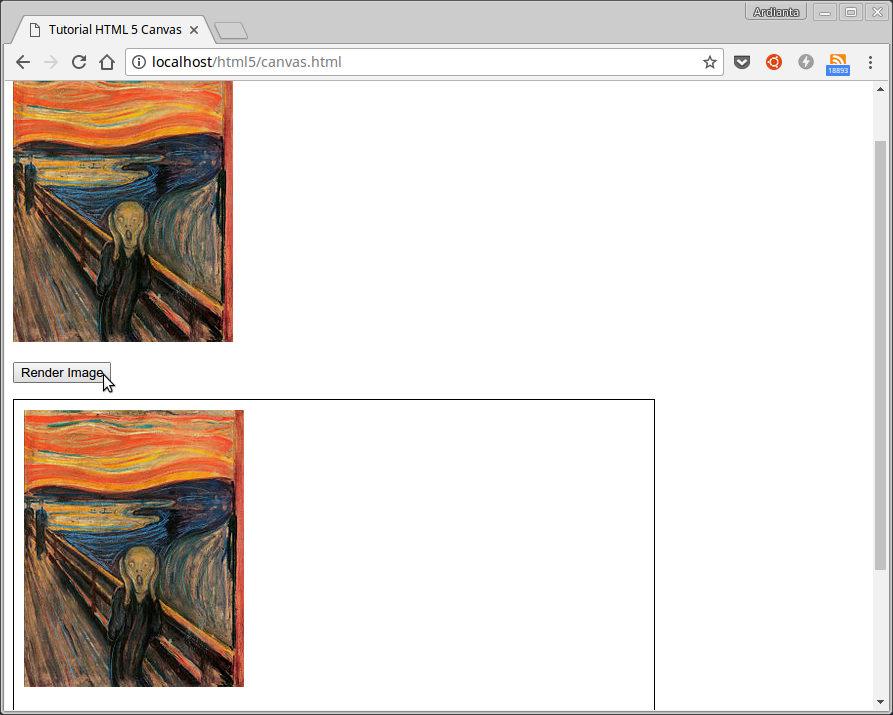Easy isn't it ...?

## HTML5 Based Game Programming

The above knowledge is not enough to make HTML5 based games from scratch, because it's just an introduction. We must learn other knowledge, such as:
• Make objects randomly on Canvas
• How to Make Animations on Canvas;
• How to Gravity and Apply Other Physical Elements;
• How to Play Audio for Games;
• Game logic and point system;
• Logic Code for Collision ;
• etc.
If you don't want to make all of them from scratch, use the Game Engine . Because it has been made there and we just use it.
The HTML5-based Game Engine list can be found at: html5gameengine.com

## Graphic Programming or Computer Graphics

Well, for those of you who are interested in graphic programming, you can try the following experimental ideas:
• Creating Painting Applications Based on HTML5;
• Make Photoshop yourself;
• Applying computer graphics algorithms such as line making algorithms;
• Image Processing and Computer Vision ;
• Creating Interactive Diagrams;
• Rendering Images from a Webcam to Canvas
• etc.

##### 0 Komentar untuk "Get to know HTML5 Canvas for Graphic Programming and Games"

Silahkan berkomentar sesuai artikel

Template By Kunci Dunia